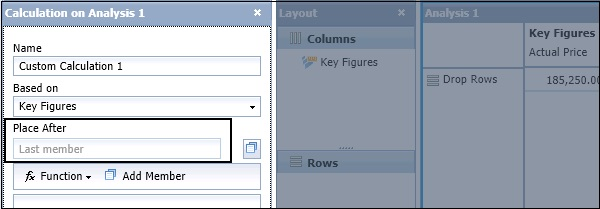# SAP BO Analysis - Calculations

In a workspace, you can add calculation to enhance the analysis and perform custom calculations in the analysis. When you add a calculation to analysis, it is used as another key figure on which you can apply Conditional Formatting and Filters.

There are different calculation types that can be used in an analysis −

### Simple Calculations

These calculations involve simple arithmetic operations - subtraction, division, percentage calculations, etc. These simple calculations can be created from the Calculation toolbar.

To add a simple Calculation, click the Calculation button in the Toolbar. This will open the Calculation panel in the task bar on the right side.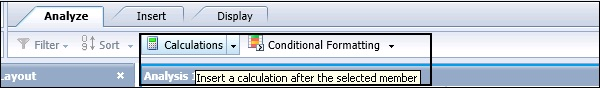In the Calculation Panel, you have to enter the name of calculation, Based on, Place After option. In calculation, you have to enter the member on which you want to perform calculation by clicking Add Member, and from Function you can select different arithmetic functions and any operand value to complete the calculation.

### Custom Calculations

Custom calculations are calculations that you define by creating formulas in the Calculation panel. Custom calculations can be as simple as an addition of two members, or can be a complex combination of functions and members.

### Dynamic Calculations

Dynamic calculations are a special type of calculation that recalculates when the data changes in its associated members.

## Create a Calculation Via Calculation Panel

When you click the Validate button, the status of expression is updated in the Calculation. To add the calculation to analysis, click the OK button.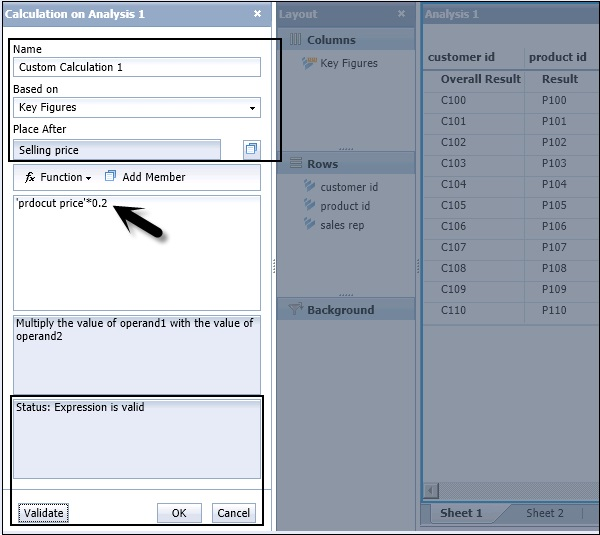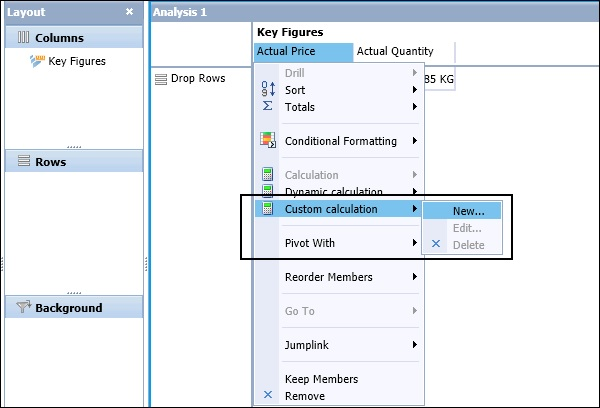To create a dynamic calculation, you have to select a key figure in the Crosstab and click the Calculation dropdown → Dynamic Calculation.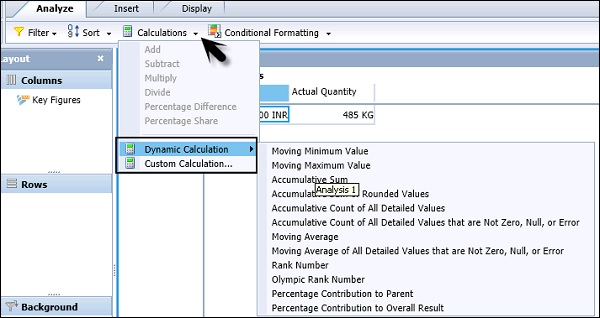You have to select one of the dynamic calculations from the list. The calculation is added as a new column or row member to the right of or beneath the selected measure. A default name is applied.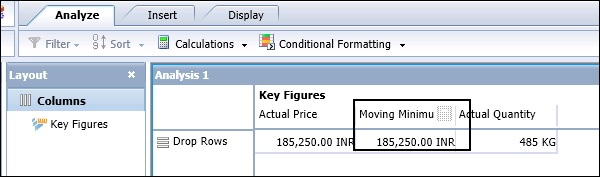Following Dynamic Calculations are available −

Dynamic Calculations Description
Moving Minimum Value Returns the current minimum value, within a hierarchy level, compared to the previous values.
Moving Maximum Value Returns the current maximum value, within a hierarchy level, compared to the previous values.
Accumulative Sum Returns the accumulative sum, within a hierarchy level, of all the previous values. This is based on the server-formatted value.
Accumulative Sum of Rounded Values Returns the accumulative sum, within a hierarchy level, of all the previous values. This is based on the displayed values.
Accumulative Count of All Detailed Values Returns the accumulative count, within a hierarchy, of all previous values.
Accumulative Count of All Detailed Values that are Not Zero, Null, or Error Returns the accumulative count, within a hierarchy, of all the previous values that are not 0, null, or error values.
Moving Average Returns the moving average, within a hierarchy, of the previous values.
Moving Average of All Detailed Values that are Not Zero, Null, or Error Returns the moving average, within a hierarchy, of the previous values that are not 0, null, or error values.
Rank Number Returns the rank order for each value, with the largest value having a rank of 1. Items with the same value will have the same rank.

Note − You can’t use dynamic calculations as operand in other calculations and filters can’t be applied on custom calculations.

## SAP BO Analysis ─ Calculation With Sorts

In an Analysis, when you create a new calculation it is added after a particular member. When you select a member in Crosstab, right-click to navigate to Calculation button and create a new calculation. The Place After field is automatically populated.

If you don’t select any member in the Crosstab and perform a Calculation, the Place After field is empty. The calculation is added to analysis and when a sort is applied, it is also sorted with the key figures.